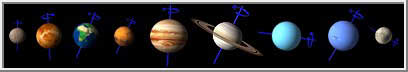# Integers and Science

Exploring Integers and Scientific Facts#### Introduction

There are many connections between integers and science. The boiling and freezing point of liquids, the melting and freezing points of solids, and the temperature of planets, are all expressed as integers. You have been selected to explore these connections.

To prepare for these explorations, you will need to browse our lessons on integers, completing the interactive exercises at the end of each. You will then complete a worksheet on integers and scientific facts. Next, you will extend your knowledge by searching on the web for additional scientific facts similar to the ones from the worksheet. Lastly, you will use the facts you gathered to create original problems for your own worksheet. These tasks will require a computer, access to the web, paper and pencil.

#### The Process

Process Part I: Learning About Integers

1. Browse our Unit on Integers, which includes Integer Properties and Arithmetic Operations.
2. Complete the five interactive exercises at the end of each lesson.

Process Part II: Apply Your Knowledge

1. Print and complete our Worksheet on Integers and Science (PDF).
2. Be sure to include the proper units for each answer.
3. Share your completed worksheet with a classmate or family member.
4. Print the answer key for the worksheet above.

Process Part III: Extend Your Knowledge

1. Search the web for ten additional scientific facts similar to the ones from our worksheet in Part II.
2. Use the facts you gathered to create ten original problems involving integers for your own worksheet.
3. Work out each problem on your worksheet to create an answer key.
4. Have a classmate or family member complete your worksheet

#### Resources

1. Math Goodies Unit on Integers.
2. Math Goodies Worksheet on Integers and Science (PDF).
3. Math Goodies answer key for the worksheet above (PDF).
4. Search engines such as BingGoogle, and Yahoo!

#### Evaluation

1. Print the answer key for our worksheet from Process Part II.